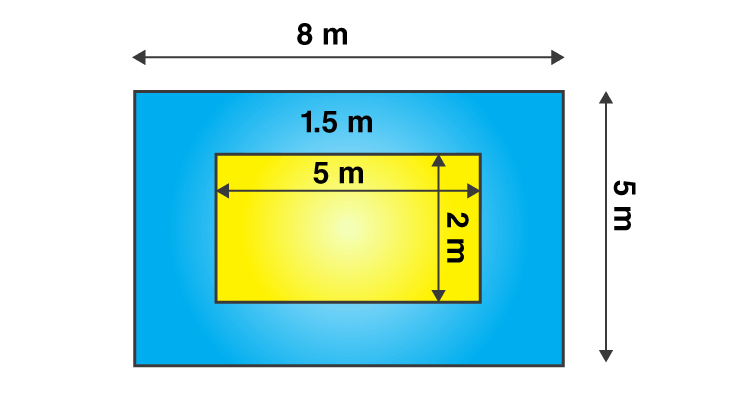Jet Set Go! All about Aeroplanes Jet Set Go! All about Aeroplanes

# Applications of area and perimeter

Applications of area and perimeter can be seen widely in our everyday life. We can observe various rectangular parks, square plots, triangular grounds, etc., that have areas and perimeters. These parameters can be determined with the help of formulas.

Not only the area and perimeter of a field but also the area and perimeter of cross paths or footpaths that surround the field can be determined by the applications of area and perimeter. Let us see some examples in this article where the formulas of area and perimeter for different shapes are applied. But before we proceed, we should know about the area and perimeter.

## Area and Perimeter of Triangle, Square and Rectangle

The perimeter of a closed figure is the distance around it. It is the total distance of boundaries of a two-dimensional figure. Thus, in general, the perimeter of any closed shape is equal to the sum of its sides. The formulas for perimeter of triangle, square and rectangle are:

 Perimeter of triangle Sum of three sides Perimeter of Square 4 x side Perimeter of rectangle 2 (length + width)

Area is the region occupied or enclosed by a two-dimensional figure in a plane. The formulas for the area of triangle, square and rectangle are:

 Area of triangle ½ (Base) (Height) Area of square Side x Side Area of rectangle Length x Width

## Solved Examples

Q.1: Find the total distance around the rectangular field if the length of the field is 50 meters and the width is 30 meters. Also, find the area of the field.

Solution: As per the given question,

The length of the field = 50 meters

The width of the field = 30 meters

By the formula of perimeter of a rectangular field, we know that the total distance around the field will be,

Perimeter = 2 (Length + Width)

= 2 (50 + 30) meters

= 2 x 80 meters

= 160 meters

Area of rectangular field = Length x width

= 50 x 30 meter2

= 1500 meter2

Q.2: A rectangular ground is 8 m long and 5 m wide such that there is a margin of 1.5 m along each of its sides. Find the total area of the margin.

Solution: As per the given question,

The length of the rectangular ground = 8 m

The breadth of the rectangular ground = 5 m

Then,

The area of the rectangular ground = length × breadth

= 8 × 5

= 40 m2From the above figure, new length and breadth of the ground when the margin of 1.5 m is not included are:

Length = 8 – (1.5 + 1.5) = 5 m

Breadth = 5 – (1.5 + 1.5) cm = 2 m

New area of the ground = 5m × 2m = 10 m2

Therefore,

Area of margin = Area of the rectangular ground when margin is included – Area of the ground when margin is not included

= 40 – 10

= 30 cm2

Hence, the required area is 30 sq.cm.

### Practice Questions

1. Find the perimeter of the greeting card that has a length of 6 inches and a breadth of 4 inches.
2. A 2 m wide path runs outside and around a rectangular park of length 100 m and breadth 70 m. Find the area of the path.
3. The area of a right triangle is 54 cm2. If one of its legs is 12 cm long, its perimeter is?

## Frequently Asked Questions on Area and Perimeter Applications

### What are the applications of area and perimeter in everyday life?

Applications of area and perimeter can be seen in everyday life, such as finding the floor area of the house, the area of the footpath that will surround the ground, fencing the park with a wire, etc.

### What is the application of perimeter?

The perimeter of a shape is the total distance covered by its sides. Thus, to find the total length of a shape, we use the perimeter formula.

### What is the application of area?

The area is the region enclosed by a shape. Thus, to find areas of a shape such as a triangle, square or rectangle, we have to use the formula.

### What is the distance around a circle called?

A circle is a closed-curved shape. The distance around the circle is called its circumference. The formula for the circumference of a circle is 2πr.

### What are some real-life applications of the area?

Floor covering using tiles or carpets, decoration of walls with paints, and installation of cupboards in a room require measurement of the area of the floors and walls, respectively.

Test your Knowledge on Applications of Area and Perimeter